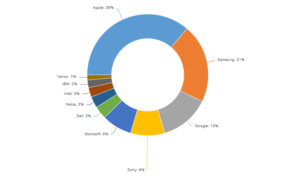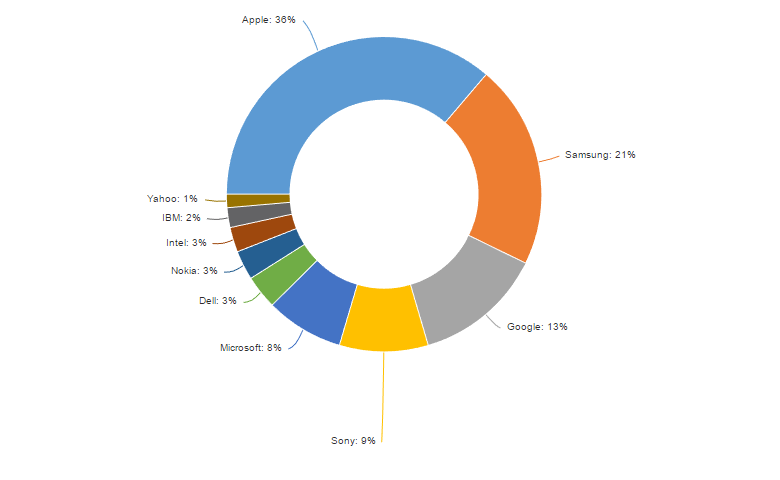Want to share your content on R-bloggers? click here if you have a blog, or here if you don't.This post discusses a number of options that are available in R for analyzing data from max-diff experiments, using the package flipMaxDiff. For a more detailed explanation of how to analyze max-diff, and what the outputs mean, you should read the post How max-diff analysis works.

The post will cover the processes of installing packages, importing your data and experimental design, before discussing counting analysis and the more powerful, and valid, latent class analysis.

# Step 1: Installing the packages

The first step is to install the flipMaxDiff package and a series of dependent packages. Depending on how your R has been setup, you may need to install none of these (e.g., if using Displayr), or even more packages than are shown below.

```
install.packages("devtools")
library(devtools)
install.packages("AlgDesign")
install_github("Displayr/flipData")
install_github("Displayr/flipTransformations")
install.packages("Rcpp")
install.packages("foreign")
install_github("Displayr/flipMaxDiff")
install_github("Displayr/flipStandardCharts")
```

# Step 2: Bring in your data

The mechanism by which you import your survery data will depend upon its format and location. For the example that is used in our main post on analysing max-diff, you can use the following to import the SPSS file:

```tech.data = foreign::read.spss("http://wiki.q-researchsoftware.com/images/f/f1/Technology_2017.sav", to.data.frame = TRUE)
```

# Step 3: Bring in the experimental design

The flipMaxDiff package contains a method for creating your own experimental design, and this is discussed more here. In many cases, you may simply need to load in the design from your PC, or from a URL. The example that we cover here uses the following:

```tech.design = read.csv("http://wiki.q-researchsoftware.com/images/7/78/Technology_MaxDiff_Design.csv")
```

# Step 4: Counting analysis

While the latent class analysis technique described below is more valid and powerful than analyses based on counting the selections made by respondents, the following code may be used to examine the results discussed in the post How max-diff analysis works.

First, collect the sections of the data set for the selections made by the respondents:

```best = tech.data[, c("Q5a_left", "Q5b_left", "Q5c_left",
"Q5d_left", "Q5e_left", "Q5f_left")]
worst = tech.data[, c("Q5a_right", "Q5b_right", "Q5c_right",
"Q5d_right", "Q5e_right", "Q5f_right")]
```

Here, the variable naming convention arises from the most-preferred option being shown on the left in the questionnaire,  and the least-preferred option being shown on the right.

This code will count up the number of times each alternative was selected as best:

```b = unlist(best) # Turning the 6 variables into 1 variable
t = table(b) # Creating a table
s = sort(t, decreasing = TRUE) # Sorting from highest to lowest
# Putting a name at the top of the column, and naming it.
best.score = cbind(Best = s)
```

This code will count up the number of selections for each of the alternatives in your max-diff experiment:

```b = table(unlist(best))
best.and.worst = cbind(Best = b,
Worst = table(unlist(worst)))[order(b, decreasing = TRUE),]
```

To compute the difference between the best and worst counts:

```diff = best.and.worst[, 1] - best.and.worst[, 2]
cbind("Best - Worst" = diff)
```

# Step 5: Latent class analysis

The flipMaxDiff package contains a function for estimating the shares in the max-diff experiment that uses latent class analysis. For a more detailed explanation of what this means, and how to interpret the outputs, see this post.

To run the latent class analysis, you can use the following:

```library(flipMaxDiff)
# Name the alternatives in the design
alt.names <- c("Apple", "Microsoft", "IBM", "Google", "Intel",
"Samsung", "Sony", "Dell", "Yahoo", "Nokia")
result = FitMaxDiff(design = tech.design,
version = rep(1, nrow(best)),
best = best,
worst = worst,
alternative.names = alt.names,
n.classes = 5,
output = "Classes")
print(result)
```

The arguments that have been used in this example are:

• design: a data frame containing the experimental design.
• version: a vector of integers which can be used to specify which respondents were shown different versions of the experimental design. In this example, the design is simple and there is only a single version shown to all respondents.
• best: a data frame containing the options that were chosen as most-preferred.
• worst: a data frame containing the options that were chosen as most-preferred.
• alternative.names: a vector containing the names of the alternatives that were used in the max-diff experiment. In the code above, the names of the technology companies are used.
• n.classes: the number of classes to include in the analysis. Refer to this post for how to work out how many classes to include.
• output: a string which denotes the type of output that should be displayed when the object is printed. In this case we have chosen “Classes”, and so the print will show information about the preference shares for each class identified. Change this to “Probabilities”, and the output will show the Mean Probability (%) and distribution of shares.

# Step 6: Obtaining respondent-level information

The latent class output can now be used to obtain various bits of information about the respondents. These can then be fed into later analyses:

To generate a new vector which tells you which class each respondent has been assigned to, you may use:

```flipMaxDiff::Memberships(result)
```

In the latent class model, each respondent has a certain probability of membership for each class. The Memberships() method used above assigns each respondent to the class with the highest probability. To see the probabilities

```input.maxdiff = result
probs = input.maxdiff\$posterior.probabilities
colnames(probs) = paste0('Class ', 1:ncol(probs) )
probs
```

Respondent-level preference shares:

```respondent.shares = prop.table(exp(as.matrix( (flipMaxDiff::RespondentParameters(result) ) ) ), 1)
```

# Step 7: Obtaining information from the output table

While the latent class analysis outputs are displayed in a cool HTMLWidget, the data in the class table can be also be extracted with results\$probabilities. This lets you extract parts of the table for charting or for other analyses. For example, in our 5-class solution, we can plot the preference shares from the Total column as a donut chart (from the package flipStandardCharts):

```library(flipStandardCharts)
pref.shares = result\$probabilities[, 6]
pref.shares = sort(pref.shares, decreasing = TRUE) * 100
Chart(pref.shares, type = "Donut")
```

# Step 8: Preference simulation

Here, preference simulation refers to the process of removing some alternatives from the calculated shares, and then rebasing the remaining shares to see how they adjust in the absence of the removed alternatives. Here, we first modify result\$probabilities by removing the Total column (which will be recomputed later), as well as the rows for the alternatives that we want to exclude. Then, the results are rebased to add to 100 using the prop.table function. A new Total column is computed by taking the weighted sum, where the weights are given by the class sizes contained in result\$class.sizes.

```# Remove the total column
x = result\$probabilities[, -6] * 100
# Removing Apple and Samsung
x = x[c(-1, -6),]
# Re-basing
x = prop.table(x, 2)* 100
sizes = result\$class.sizes
x = cbind(x, Total = rowSums(sweep(x, 2, sizes, "*")))
new.preferences = rbind(x, Total = colSums(x))
```

# Summary

Once you have loaded in your survey data and experimental design, the latent class analysis from flipMaxDiff can be run with a couple of lines of code. Additional methods are then available for saving respondent-level information, and data from the output table, to feed them in to your charts and analyses.